# AIRS Data Compression (Preliminary Work)

The first part of our work was determining what a good baseline would be for our compression goals. We try to answer the questions: Can the data be compressed and can we improve on current algorithms?

### Preliminary Processing

 We did not want any of our algorithms to break the dimensionality of the data so as not to disturb the natural continuity of the images. The 90x135 images represent readings from geographically adjacent (or close) locations, and the 2378 channels are readings from adjacent frequencies, so we would expect continuity along all three dimensions. The video (right) shows the 90x135 scan image as the channel varies, and supports our hypothesis.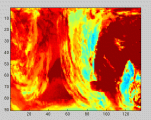(1:27 MPEG, 10.89 MB)

The data contained 51 discontinuous channels (see channel 964 for an example); we assumed that these were so called "radiometric reference channels". Our group performed a simple calculation (the square root of the integral of the squared differences between two adjacent channels) to discover these "bad" channels, and store them separately.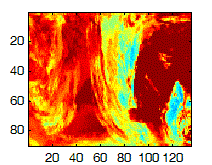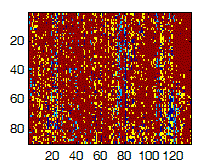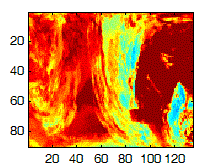### Preliminary Work

We applied a 2D Discrete Cosine Transform to the original 90x135 image, and noticed that many of the coefficients were negligible (as seen by the blue in the image below).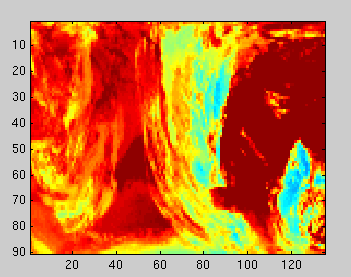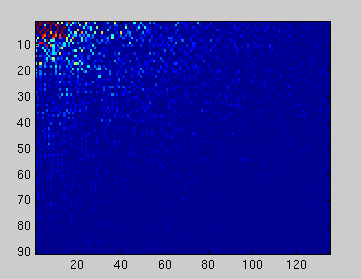After applying the discrete cosine transform to each 90x135 image in our cube, we looked at the new cube along the channels (Figure 4). Because of the structure of the image, we chose the Haar transform as a preliminary transform.Original Slice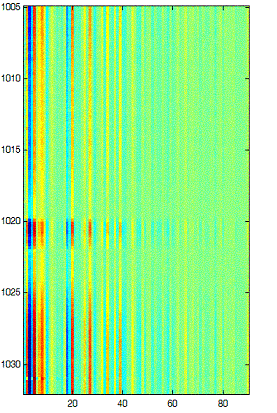Slice after 2D DCTSlice after Haar

We then used an adapted Huffman algorithm for coding of the transformed image. Due to project constraints (mostly error correction demands of NASA), our group will focus on fixed-length codes for the final product. However, Huffman is provably optimal (it is a form of entropy coding) and thus serves as a good benchmark for our future work.

### Preliminary Results

We achieved a 10:1 preliminary compression ratio (lossy).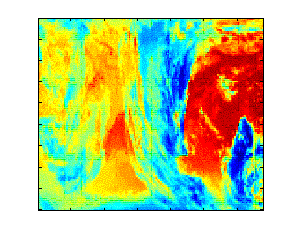Original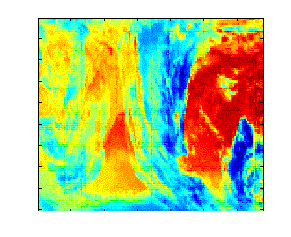Compressed<->Decompressed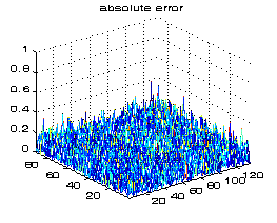Absolute Error: ± 0.2

### Ongoing Work

• Adaptive Thresholding: We use a constant threshold to remove the "bad" images. This is not suitable since the range of the data varies from one section of the spectrum to another. The impact of bad channels on the compression ratio is significant. Therefore, we will study more adaptive thresholding techniques.
• Non-separable wavelet transforms: Because of physical properties of the signal, we believe that wavelets will deliver the best transformation of the data (with respect to entropy). Many of current applications of wavelets use so-called "separable wavelet bases" which impose a structure on the plane which is artificial to most images and leads to damage that becomes obvious at the compression ratios demanded by the new hyperspectral instruments.
• Fixed-Length Coding Algorithms: The electromagnetic spectrum is unstable, and, therefore, error correction is a key issue. To minimize the impact of error correction on our compression ratio, we will be looking at non-entropy coding techniques.
• Assembly Programming: The software will reside onboard the real-time satellite system, and, therefore, must be optimized to operate in this environment. The upper bound on the entire procedure is 12 seconds during which time it must process 1 granule (6 minutes or 231.1416 MB of data). For the new GOES-R system, the data rate is 34 times greater. Therefore, some assembly will be written along key execution paths (especially in the advanced mathematical subroutines -- eigenvector calculation, etc).

### Notes

*We were provided Level 1B AIRS data. The data is shared as an HDF EOS file (this is a standard for scientific data and can be accessed via matlab's hdfsw gateway API). Each HDF file (or level 1B "granule") represents 6 minutes of data (or 135 scans for each of the 2378 channels).